# Concentric circles

There is given a circle K with a radius r = 8 cm. How large must a radius have a smaller concentric circle that divides the circle K into two parts with the same area?

Correct result:

r2 =  5.657 cm

#### Solution:

$r=8 \ \text{cm} \ \\ \ \\ S=\pi \cdot \ r^2=3.1416 \cdot \ 8^2 \doteq 201.0619 \ \text{cm}^2 \ \\ \ \\ S_{2}=S/2=201.0619/2 \doteq 100.531 \ \text{cm}^2 \ \\ \ \\ S_{2}=\pi \cdot \ r_{2}^2 \ \\ \ \\ r_{2}=\sqrt{ S_{2}/\pi }=\sqrt{ 100.531/3.1416 }=4 \ \sqrt{ 2 }=5.657 \ \text{cm}$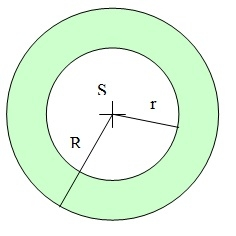Our examples were largely sent or created by pupils and students themselves. Therefore, we would be pleased if you could send us any errors you found, spelling mistakes, or rephasing the example. Thank you!

Please write to us with your comment on the math problem or ask something. Thank you for helping each other - students, teachers, parents, and problem authors.#### You need to know the following knowledge to solve this word math problem:

We encourage you to watch this tutorial video on this math problem:

## Next similar math problems:

• Ratio of squaresA circle is given in which a square is inscribed. The smaller square is inscribed in a circular arc formed by the side of the square and the arc of the circle. What is the ratio of the areas of the large and small squares?
• Truncated cone 6Calculate the volume of the truncated cone whose bases consist of an inscribed circle and a circle circumscribed to the opposite sides of the cube with the edge length a=1.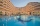Up to 48 rooms, some of which are triple and some quadruple, accommodated 173 people so that all beds are occupied. How many triple and how many quadruple rooms were there?
• Sow barley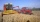Farmers wanted to sow barley within 13 days. Due to the excellent weather, they managed to exceed the daily plan of sowing by 2 ha and therefore finished sow grain in 12 days. How many hectares of land did they sow with barley?
• Volume ratio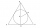Calculate the volume ratio of balls circumscribed (diameter r) and inscribed (diameter ϱ) into an equilateral rotating cone.
• The regularThe regular triangular prism has a base in the shape of an isosceles triangle with a base of 86 mm and 6.4 cm arms, the height of the prism is 24 cm. Calculate its volume.
• Company carMs. Vaňková has a company car equipped with a multifunction indicator, on which she can monitor the average petrol consumption during individual rides. During the first ride, mostly in city traffic, it traveled 20.5 km, with an average consumption of 7.8L
• The funnel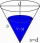The funnel has the shape of an equilateral cone. Calculate the content of the area wetted with water if you pour 3 liters of water into the funnel.
• Equilateral coneWe pour so much water into a container that has the shape of an equilateral cone, the base of which has a radius r = 6 cm, that one-third of the volume of the cone is filled. How high will the water reach if we turn the cone upside down?
• Big numbersHow many natural numbers less than 10 to the sixth can be written in numbers: a) 9.8.7 b) 9.8.0
• Hexagonal pyramidFind the area of a shell of the regular hexagonal pyramid, if you know that its base edge is 5 cm long and the height of this pyramid is 10 cm.
• Triangular prismThe triangular prism has a base in the shape of a right triangle, the legs of which is 9 cm and 40 cm long. The height of the prism is 20 cm. What is its volume cm3? And the surface cm2?
• ParallelogramFind the perimeter of the parallelogram, where base a = 8 cm, height v = 3 cm, and angle alpha = 35° is the magnitude of the angle at vertex A.
• What is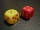What is the probability that the sum of 9 will fall on a roll of two dice? Hint: write down all the pairs that can occur as follows: 11 12 13 14 15. . 21 22 23 24. .. . 31 32. .. . . . . . .. . 66, count them, it's the variable n variable m: 36, 63,. .. .
• Quarantine cupcakes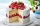Mr. Honse was baking quarantine cupcakes. Mrs. Carr made twice as many as Mr. Honse. Ms. Sanchez made 12 cupcakes more than Mr. Honse. If they put all their cupcakes together (which they can’t because. .. quarantine!) they would have 108 cupcakes. How may
• 2 cyclists and carOne cyclist rides at a constant speed over a bridge. It is 100 meters long. When he is 40 meters behind him, he meets an oncoming cyclist who is riding at the same speed. The car travels along the bridge in the same direction as the first cyclist at a spe
• Lottery - eurocentsTereza bets in the lottery and finally wins. She went to the booth to have the prize paid out. An elderly gentleman standing next to him wants to buy a newspaper, but he is missing five cents. Tereza is in a generous mood after the win, so she gives the m